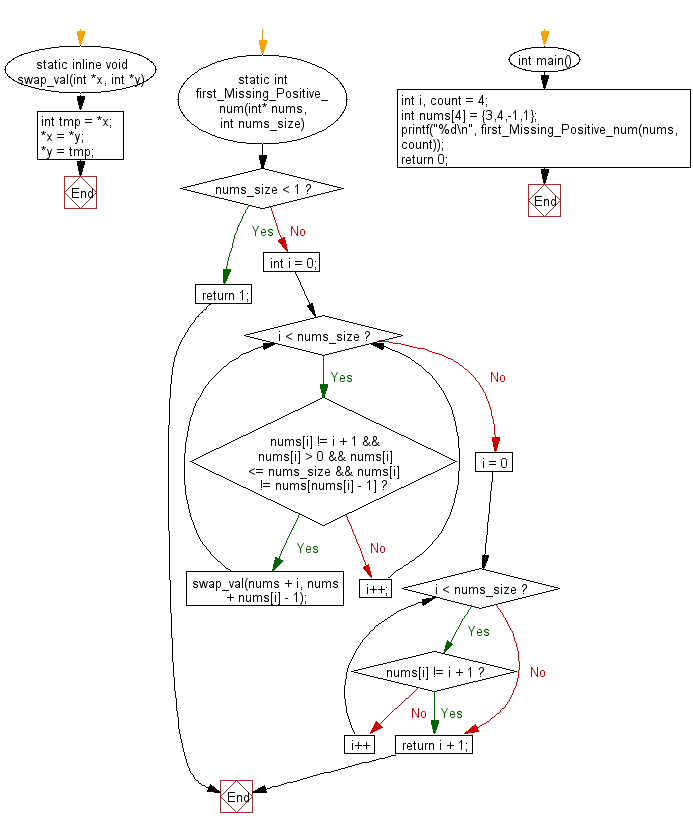﻿ C Program: First missing +ve integer in unsorted array# C Exercises: Find the first missing positive integer from a given unsorted integer array

## C Programming Practice: Exercise-28 with Solution

Write a C programming to find the first missing positive integer from a given unsorted integer array.

C Code:

``````//https://github.com/begeekmyfriend/leetcode/blob/master/041_first_missing_positive/missing_positive.c
#include <stdio.h>
#include <stdlib.h>
static inline void swap_val(int *x, int *y)
{
int tmp = *x;
*x = *y;
*y = tmp;
}

static int first_Missing_Positive_num(int* nums, int nums_size)
{
if (nums_size < 1) {
return 1;
}

int i = 0;
while (i < nums_size) {
if (nums[i] != i + 1 && nums[i] > 0 && nums[i] <= nums_size && nums[i] != nums[nums[i] - 1]) {
swap_val(nums + i, nums + nums[i] - 1);
} else {
i++;
}
}

for (i = 0; i < nums_size; i++) {
if (nums[i] != i + 1) break;
}
return i + 1;
}

int main()
{
int i, count = 4;
int nums = {3,4,-1,1};

printf("%d\n", first_Missing_Positive_num(nums, count));
return 0;
}
``````

Sample Output:

```2
```

Flowchart:## C Programming Code Editor:

What is the difficulty level of this exercise?

Test your Programming skills with w3resource's quiz.

﻿

## C Programming: Tips of the Day

What is the difference between printf() and puts() in C?

puts is simpler than printf but be aware that the former automatically appends a newline. If that's not what you want, you can fputs your string to stdout or use printf.

Ref :https://bit.ly/3f2fwcH